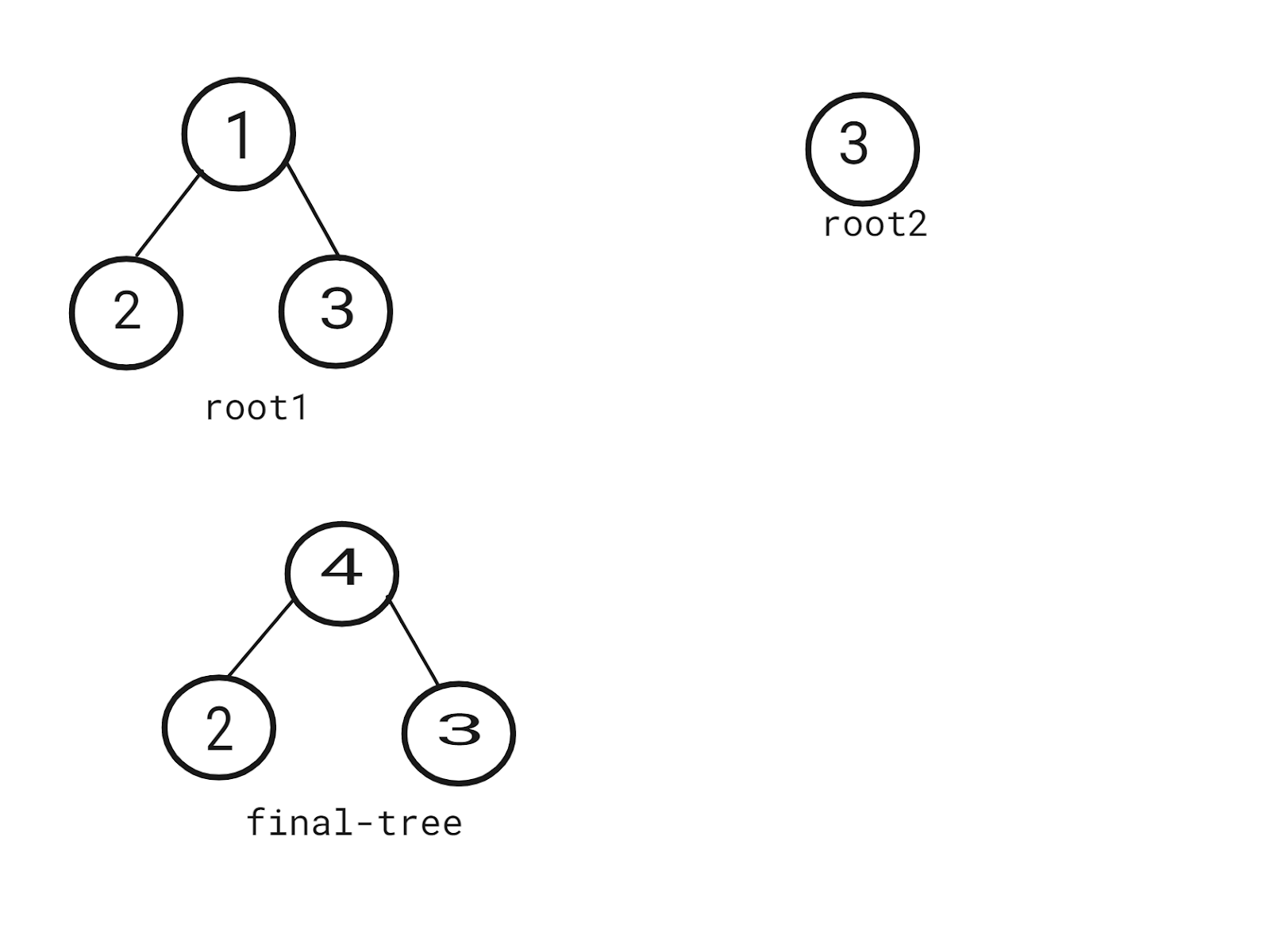# Merge Two Binary Trees

Posted: 17 Mar, 2021
Difficulty: Easy

## PROBLEM STATEMENT

#### Note:

``````The merging process must start from the root nodes of both trees.
``````

#### For example,

``````‘ROOT1’ = [1, 2, -1, -1, 3, -1, -1]  ‘ROOT2’ = [3, -1, -1].
The final tree would look like : [3, 2, -1, -1, 3, -1, -1], and the output will be printed in a pre-order way: 4 2 3.
``````#### Input format :

``````The first line of input contains an integer ‘T’ denoting the number of test cases.

In the next 2*T lines, the first line of each test case contains space-separated integers denoting the nodes of the first binary tree, where -1 indicates that the NULL pointer has been appointed to the previous node

The second line of each test case contains space-separated integers denoting the nodes of the second binary tree, where -1 indicates that the NULL pointer has been appointed to the previous node.

The input is given in a preorder way, that is, the node then left subtree, and then right subtree as shown in the example.
``````

#### Output format :

``````For each test case, return the resultant binary tree in a pre-order way.

The output of each test case will be printed in a separate line.
``````

#### Note:

``````You do not need to print anything. It has already been taken care of. Just implement the given function.
``````

#### Constraints:

``````1 <= T  <= 5
1 <= N <= 10^3
-10^3 <= DATA <= 10^3

Time Limit: 1 second
``````Approach 1

The main idea is to use recursion to traverse the tree in a Pre-order way. If any of the nodes is null, return the other node, and if both are NULL, return NULL.

• We can traverse both the given trees in a preorder fashion. We check if the current node exists for both the trees.
• We add the values in the current nodes of both the trees and update the value in the current node of the first tree to reflect this sum obtained.
• If at any step one of these children happens to be null, we return the child of the other tree(representing the corresponding child subtree) to be added as a child subtree to the calling parent node in the first tree.
• In the end, the first tree will represent the required resultant merged binary tree.